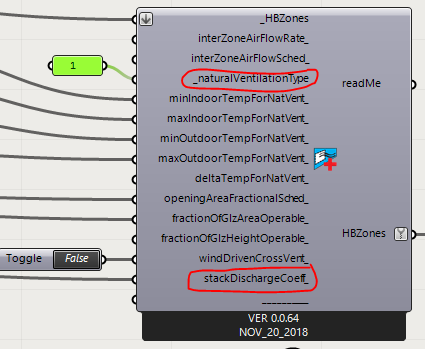# Natural Ventilation Component Formula

Hi there,

Would anyone be able to clarify whether the first formula (below) is used when we select natural ventilation type 1 in Honeybee Set EP airflow component ?

Formulas from the code are:

1. *Ventilation Wind = Wind Coefficient * Opening Area * Schedule * WindSpd *
2. Ventilation Stack = Stack Discharge Coefficient * Opening Area * Schedule * SQRT(2 * Gravity * Operable Height * (|(Temp Zone - Temp Outdoors)| / Temp Zone))I have single sided ventilation so I selected natural ventilation type 1, however, what I don’t understand is the stack discharge coefficient coming into play. If I change the stack discharge coefficient to 0, I get all zeros in the ‘wind ventilation standard density volume flow rate’ output, meaning there is no ventilation. Which means that the discharge coeffieicent has an impact even if I choose ventilation type 1.

Any idea what is the formula used when we select type 1 ventilation?

Many thanks

Yes, those are the formulas being used and you can see them in the Input/Output reference for the EnergyPlus object that is being used under the hood:

And, yes, the discharge coefficient of the windows has an impact on 1 - Window Natural Ventilation. It’s how you account for things like the shape of the window opening and/or insect screens. Why would you think it would not?

Many thanks @chris for getting back to me on this and confirming that the discharge coefficient impacts ventilation type 1.

I think I was slighlty confused thinking that if I choose natural ventilation type 1, then the component would use the first formula (ventilation wind) which doesn’t have the discharge coefficient in the variables, so I wondered how is it affecting the output. I can only see the discharge coefficient under the second formula (ventilation stack)

so is the component equation at the end combining both ventilation wind + ventilation stack as the link you shared shows when we choose type 1?

Yes, @Farah.H . That’s exactly what it’s doing. So you can account for BOTH the stack and the cross-ventilation effects of open windows.

1 Like